# The first row of squares for general k

Let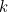be a positive integer. Look at the first row of larger squares in the complement of the blue grid you get from colouring squares corresponding to multiples ofblue. First, we are going to prove that the numbers within the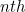larger square from the left add to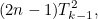where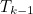is the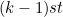triangular number.

Let’s call the square in question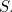First note that the numbers within the top row of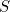are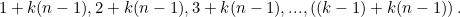Adding those up we get the sum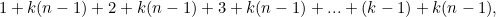which we can rearrange to give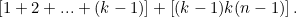(1)

As we mentioned in the main article, there’s a formula for the sum of the first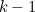integers: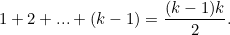(2)

Substituting this into the left part of expression (1) gives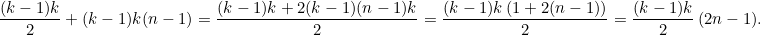(3)

Now thetriangular number is equal to the sum of the firstintegers, so it’s also equal to the right hand side of equation (2). This means that expression (3) is equal to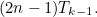Now notice that the numbers in the second row ofare 2 times the numbers in the first row, so their sum is equal to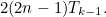The numbers in the second row ofare 3 times the numbers in the first row, so their sum is equal to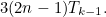We can continue in this vein until we come to the sum of the last row of, which is equal to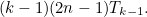Adding up the sums of all the rows intherefore gives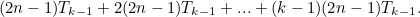(4)

Factoring out the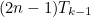transforms this sum to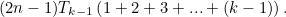(5)

Now the second bracket here is equal to, thetriangular number. Therefore our sum (5) is equal toas we claimed.

• Want facts and want them fast? Our Maths in a minute series explores key mathematical concepts in just a few words.